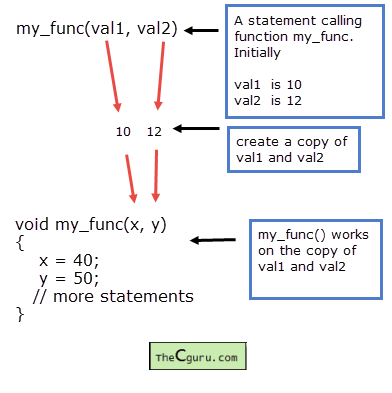# Call by Value and Call by Reference in C

Last updated on July 27, 2020

C provides two ways of passing arguments to a function.

1. Call by value or Pass by value.
2. Call by reference.

## Call by Value #

In this method a copy of each of the actual arguments is made first then these values are assigned to the corresponding formal arguments.This means that the changes made by the called function have no effect on the values of actual arguments in the calling function. In the example shown in the above figure, my_func() function modifies a copy of the values of val1 and val2. However, the original value of val1 and val2 remains the same.

All the function we have written so far uses call by value except the ones in which we passed an array to the function.

## Call by reference #

In this method addresses of the actual arguments are copied and then assigned to the corresponding formal arguments. Now formal and actual arguments both points to the same data (because they contain the same address). As a result, any changes made by called function also affect the actual arguments.

Let's take some examples:

The following program demonstrates call by value:

  1 2 3 4 5 6 7 8 9 10 11 12 13 14 15 16 17 18 19 20 21 22 23 24 25 26 27 28 29 30 31 #include void try_to_change(int, int); int main() { int x = 10, y = 20; printf("Initial value of x = %d\n", x); printf("Initial value of y = %d\n", y); printf("\nCalling the function\n"); try_to_change(x, y); printf("\nValues after function call\n\n"); printf("Final value of x = %d\n", x); printf("Final value of y = %d\n", y); // signal to operating system program ran fine return 0; } void try_to_change(int x, int y) { x = x + 10; y = y + 10; printf("\nValue of x (inside function) = %d\n", x); printf("Value of y (inside function) = %d\n", y); } 

Expected Output:

  1 2 3 4 5 6 7 8 9 10 Initial value of x = 10 Initial value of y = 20 Value of x (inside function) = 20 Value of y (inside function) = 30 Values after function call Final value of x = 10 Final value of y = 20 

How it works:

The variables x and y inside function main() and variable x and y in the formal arguments of function try_to_change() are completely different. In line 13, when try_to_change() function is called a copy of values of x and y is made and that copy is passed to the formal arguments x and y of the function try_to_change(). Inside the function try_to_change() we have tried to change the original value of x and y by assigning new values to it. Since try_to_change() is working on a copy of x and y, changes made by try_to_change() function will have no effect on the actual arguments x and y.

To use call by reference we need to do two things:

1. Pass the addresses of the actual arguments instead of passing values to the function.
2. Declare the formal arguments of the function as pointer variables of an appropriate type.

The following program demonstrates call by reference.

  1 2 3 4 5 6 7 8 9 10 11 12 13 14 15 16 17 18 19 20 21 22 23 24 25 26 27 28 29 30 31 #include void try_to_change(int *, int *); int main() { int x = 10, y = 20; printf("Initial value of x = %d\n", x); printf("Initial value of y = %d\n", y); printf("\nCalling the function\n"); try_to_change(&x, &y); printf("\nValues after function call\n\n"); printf("Final value of x = %d\n", x); printf("Final value of y = %d\n", y); // signal to operating system everything works fine return 0; } void try_to_change(int *x, int *y) { (*x)++; (*y)++; printf("\nValue of x (inside function) = %d\n", *x); printf("Value of y (inside function) = %d\n", *y); } 

Expected Output:

  1 2 3 4 5 6 7 8 9 10 11 12 Initial value of x = 10 Initial value of y = 20 Calling the function Value of x (inside function) = 11 Value of y (inside function) = 21 Values after function call Final value of x = 11 Final value of y = 21 

Here we are passing addresses of integer variables to a function. So the formal arguments must be declared as a pointer to int or (int *). The expression (*x)++ means that first dereference the value at x then increment it. Similarly, (*y)++ means that first dereference the value at y then increment it. When the function try_to_change() ends, the control passes back to main() and printf() statements in line 17 and 18 prints the new value of x and y respectively.